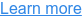# Power vs Energy: What's the Difference?

Posted on Monday Jun 29, 2020 at 01:30PM

Often when customers say, “I just want to monitor power” they really mean ‘Real Power’ or ‘Energy.’

## Power vs Energy

Power and energy are closely related, but they are not interchangeable. Simply put, power is the rate at which work is done. While energy is the capacity to do work.

## What is Power

Before we tackle energy versus demand, let’s take a moment to first discuss power, since power is at the root of all three terms. In an alternating current (AC) power application, there are three types of power available:

1. Apparent Power
2. Real Power
3. Reactive Power

Apparent Power is simply the voltage multiplied by the amperage.  It is primarily used to determine the size of conductors (wires, bus bars) and transformers.  The Apparent Power is the total power available for use.

Real Power is a ‘snapshot’ of the power needed and put to use at a given time….It can change from moment to moment.  The Power Factor (PF) is needed to calculate the Real Power in an AC system.

## P = I EL-L(PF)

P = Real Power, measured in Watts, (W)

I = Current, measured in Amperage, (A)

E = Voltage, measured in Volts, (V) {Line-to-Line}

PF = Power Factor is an expression of the efficiency of a load.  How much of the power supplied is actually doing real work?  PF has a range of 0.0 to 1.0.  An ideal system has a PF of 1, meaning all of the power supplied is doing real work.  PF values less than 0.7 are considered to be a poor use of power and a utility may charge penalty fees for wasting power.

The third type of power, Reactive Power (kVAR) is the power demanded by inductive or capacitive loads.  Motors and PC power supplies are examples of reactive loads that can increase Reactive Power.  An increase in Reactive Power has a negative effect on the PF. Some end users want to monitor Reactive Power to better manage their overall PF and / or the negative effects on PF caused by specific loads. Power Factor Correction Capacitors (PFCCs) can be used to improve the PF of specific loads or systems. The illustration below shows how the three types of power are mathematically related. As you can see in this illustration, as the reactive power decreases the real and apparent powers come closer and closer to being equal (unity).Here’s another way to think of the relationship between Real, Apparent Power, and Reactive Power…. ☺The beer above isn’t a particularly good pour, but hopefully you get the gist. The beer you will actually drink is the Real Power. The foam, the wasted beer, is the Reactive Power. The entire volume of the beer poured is the Apparent Power.

Now that you have an understanding of power, we must add another element to calculate Energy and Demand. That element is time.

Energy is the amount of power consumed over a period of time. It is measured in kilowatt hours.

Demand is the average amount of power (kW) used for a given time period. In power metering applications, customers who want monitor Demand (or kW Demand) must select a demand time interval. This time interval can be five, ten, or fifteen minutes or whatever interval the end user wants to capture for future use. The time interval setting is critical to calculating the average power.

## Power vs Energy: Summary

Power usage will fluctuate over time. For our discussion today, let’s look at a simple graph of a pretty steady use of power over time.Power or Real Power (kW) is a snapshot. It is one data point on our graph.

Energy (kWh) is the total power used over a period of time.

Demand (kW Demand) is the average power used over a period of time.## Indoor Air Quality Monitors

Comply with indoor air quality standards for healthy and safe building environments